Courses

# RD Sharma Test: Statistics

## 25 Questions MCQ Test Mathematics (Maths) Class 10 | RD Sharma Test: Statistics

Description
This mock test of RD Sharma Test: Statistics for Class 10 helps you for every Class 10 entrance exam. This contains 25 Multiple Choice Questions for Class 10 RD Sharma Test: Statistics (mcq) to study with solutions a complete question bank. The solved questions answers in this RD Sharma Test: Statistics quiz give you a good mix of easy questions and tough questions. Class 10 students definitely take this RD Sharma Test: Statistics exercise for a better result in the exam. You can find other RD Sharma Test: Statistics extra questions, long questions & short questions for Class 10 on EduRev as well by searching above.
QUESTION: 1

### In the given data if n = 230, l = 40, cf = 76, h = 10, f = 65, then its median is

Solution:

Median=L +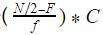=40 +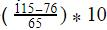=40 + 6 = 46

QUESTION: 2

### In a data, if l = 40, h = 15, f1=7, f0=3, f2=6, then the mode is

Solution:

Mode=L+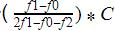Where L=lower limit of the modal class =40
f1= modal class frequency.=7
f2 = just after the modal class frequency.=6
f0 = just previous the modal class frequency=3
C = Class size=15
Mode=40+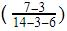* 15 = 40 + 12 = 52

QUESTION: 3

### The mode of 4, 5, 6, 8, 5, 4, 8, 5, 6, x, 8 is 8. The value of ‘x’ is

Solution:

Mode is the most occurred value. In the data we have three 5 and three 8 , so if mode is only 8 then the value of x must be 8 ,so that only 8 is the most occurring value.

QUESTION: 4

The wickets taken by a bowler in 10 cricket matches are 2, 6, 4, 5, 0, 3, 1, 3, 2, 3. The mode of the data is

Solution:

Mode is the most frequently occurring observation. Since it's not a grouped data the frequency is the occurrence of only one observation , So the highest no. of frequency is 3 . So the modal age is 3.

QUESTION: 5

In a data, if l = 60, h = 15, f= 16, f= 6, f= 6, then the mode is

Solution:
QUESTION: 6

The mode of 4, 5, 6, 8, 5, 4, 6, 5, 6, x, 8 is 6. The value of ‘x’ is

Solution:
QUESTION: 7

For the following distribution the lower limit of the modal class is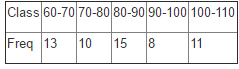Solution:
QUESTION: 8

For the following distribution the modal class is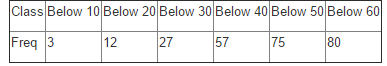Solution:

Modal class as the name suggests is the interval which occurs most of the times which means the interval which has the highest frequency.By changing the distribution in grouped distribution 30-40 has the maximum frequency that is 30

QUESTION: 9

The times, in seconds, taken by 75 athletes to run a 500m race are tabulated as below: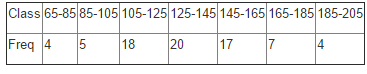The number of athletes who completed the race in less than 125 seconds is

Solution:
QUESTION: 10

The median of first 10 prime numbers is

Solution:

A prime number is a natural number greater than 1 that is not a product of two smaller natural numbers. So 10 prime numbers are: 2,3,5,7,11,13,17,19,23,29
Since the number of terms are even ,
Median =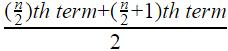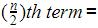10/2=5th term=11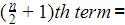10/2 +1=6th term=13
Median =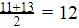QUESTION: 11

The most frequent value in the data is known as

Solution:
QUESTION: 12

The marks obtained by 9 students in Mathematics are 59, 46, 30, 23, 27, 44, 52, 40 and 29. The median of the data is

Solution:
QUESTION: 13

The marks obtained by 9 students in Mathematics are 59, 46, 30, 23, 27, 40, 52, 35 and 29. The median of the data is

Solution:
QUESTION: 14

The percentage of marks obtained by 100 students in an examination are as follows: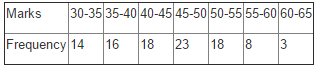The median class is

Solution:
QUESTION: 15

In the given data if n = 44, l = 400, cf = 8, h = 100, f = 20, then its median is

Solution:
QUESTION: 16

The measure of central tendency that can be obtained graphically is

Solution:

Mode is the value with the maximum frequency. Thus, it can be determined from the graph.
Median is the middle value of the data. Thus, it can be determined from the graph.
Mean is the ratio of sum of all data values and the total number of values. Thus, it cannot be determined by graphically.

QUESTION: 17

The wickets taken by a bowler in 10 cricket matches are 2, 6, 4, 5, 0, 3, 1, 3, 2, 3. The median of the data is

Solution:
QUESTION: 18

The marks obtained by 9 students in Mathematics are 59, 46, 30, 23, 27, 44, 52, 40 and 29. The median of the data is

Solution:

Median is the middle value of the observations. So Median cannot be obtained when the observations are not in order. So first we have to arrange in ascending order.
59, 46, 30, 23, 27, 44, 52, 40 and 29
Gives- 23,27,29,30,40,44,46,52,59
Since the no. of observations is odd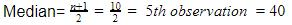QUESTION: 19

In the given data if n = 230, l = 40, cf = 76, h = 10, f = 65, then its median is

Solution:
QUESTION: 20

Construction of cumulative frequency table is useful to determine

Solution:
QUESTION: 21

The middle most value of the data is

Solution:
QUESTION: 22

The abscissa of the point of intersection of the less than type and of the more than type ogives of a grouped data gives its

Solution:
QUESTION: 23

An ‘ogive’ is used to determine

Solution:

An give, also known as a cumulative histogram, is a graph that is used to determine the number of data points that are equal to or below a certain value in a data set.It is a plot of cumulative values, rather than a plot of the values themselves. Median is determined by finding the (n/2)th value and then dropping it to the x axis.

QUESTION: 24

To represent ‘the less than type’ graphically, we plot the ____________ on the x – axis.

Solution:
QUESTION: 25

To represent ‘the more than type’ graphically, we plot the ____________ on the x – axis.

Solution: